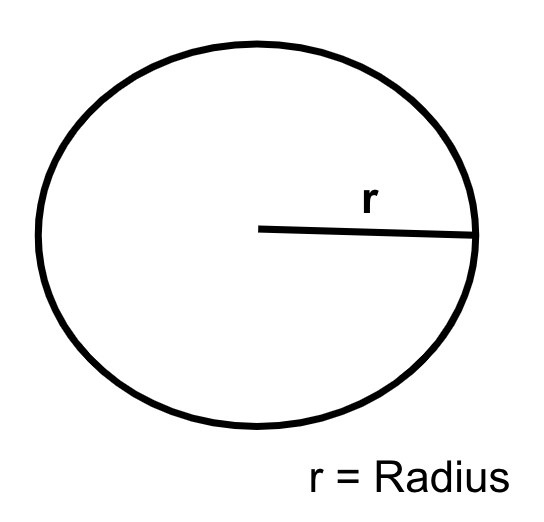# How to Find the Perimeter of a Circle in Golang?

In this tutorial, we are going to see the Golang program to find the perimeter of a Circle. Perimeter is the total length of the boundary of any closed figure.## Formula

Perimeter of Circle - 2 * 22 / 7 * r
r - radius of a Circle


For example, the radius of a Circle is 10 cm so the perimeter of a Circle is −

perimeter = 2 * 22 / 7 * 10
= 62.8571428


## Finding the perimeter of a circle within the function

### Algorithm

Step 1 − Declaring the variables for the radius and the perimeter of the float64 data type.

Step 2 − Initialize the variable radius with the respective value.

Step 3 − Find the perimeter using the above formula within the function.

Step 4 − Printing the result.

Time Complexity:
O(1)

Space Complexity:
O(1)


## Example 1

In this example, we are going to find the Perimeter of a Circle within the function.

package main
// fmt package provides the function to print anything
import (
"fmt"
)
func main() {
// declaring the floating variables using the var keyword for
// storing the radius of the circle also a variable perimeter to store perimeter
fmt.Println("Program to find the perimeter of a Circle.")

// initializing the radius of a circle

// finding the perimeter of a Circle using the formula
// and stored in the perimeter variable of float64 type
perimeter = 2 * (22 / 7.0) * radius

// printing the result
fmt.Println("The perimeter of a Circle whose radius is", radius, "=", perimeter, "cm.")
fmt.Println("(Finding the perimeter of a circle within the function)")
}


## Output

Program to find the perimeter of a Circle.
The perimeter of a Circle whose radius = 5 is 31.428571428571427 cm.
(Finding the perimeter of a circle within the function)


## Description of code

• var radius, perimeter float64 − In this line, we are declaring the radius and perimeter that we are going to use later. As the radius or perimeter can be in decimal, we have used the float data type.

• perimeter = 2 * (22 / 7.0) * radius − In this line of code, we are applying the formula and finding the perimeter.

• fmt.Println("The perimeter of a Circle whose radius is", radius, "is", perimeter, "cm.")

- Printing the perimeter of a Circle.

## Finding the perimeter of a circle in the separate function

### Algorithm

Step 1 − Declaring the variables for the radius and the perimeter of the float64 data type.

Step 2 − Initialize the variable radius with the respective value.

Step 3 − Calling the function with the radius as a perimeter, and storing the perimeter that the function is returning.

Step 4 − Printing the result.

## Example 2

In this example, we are going to find the perimeter of a Circle by defining the separate function to find the perimeter.

package main
// fmt package provides the function to print anything
import (
"fmt"
)

// function has a perimeter of float64 type and has a return type of loat64
// returning the perimeter by applying the formula
return 2 * (22 / 7.0) * radius
}
func main() {
// declaring the floating variables using the var keyword for
// storing the radius of the circle also a variable perimeter to store perimeter
fmt.Println("Program to find the perimeter of a Circle.")

// initializing the radius of a circle

// finding the perimeter of a Circle using a separate function

// printing the result
fmt.Println("The perimeter of a Circle whose radius is", radius, "is", perimeter, "cm.")
fmt.Println("(Finding the perimeter of a circle in the separate function)")
}


## Output

Program to find the perimeter of a Circle.
The perimeter of a Circle whose radius = 5 is 31.428571428571427 cm.
(Finding the perimeter of a circle in the separate function)


## Description of code

• var radius, perimeter float64 − In this line, we are declaring the radius and perimeter that we are going to use later. As the radius or perimeter can be in decimal, we have used the float data type.